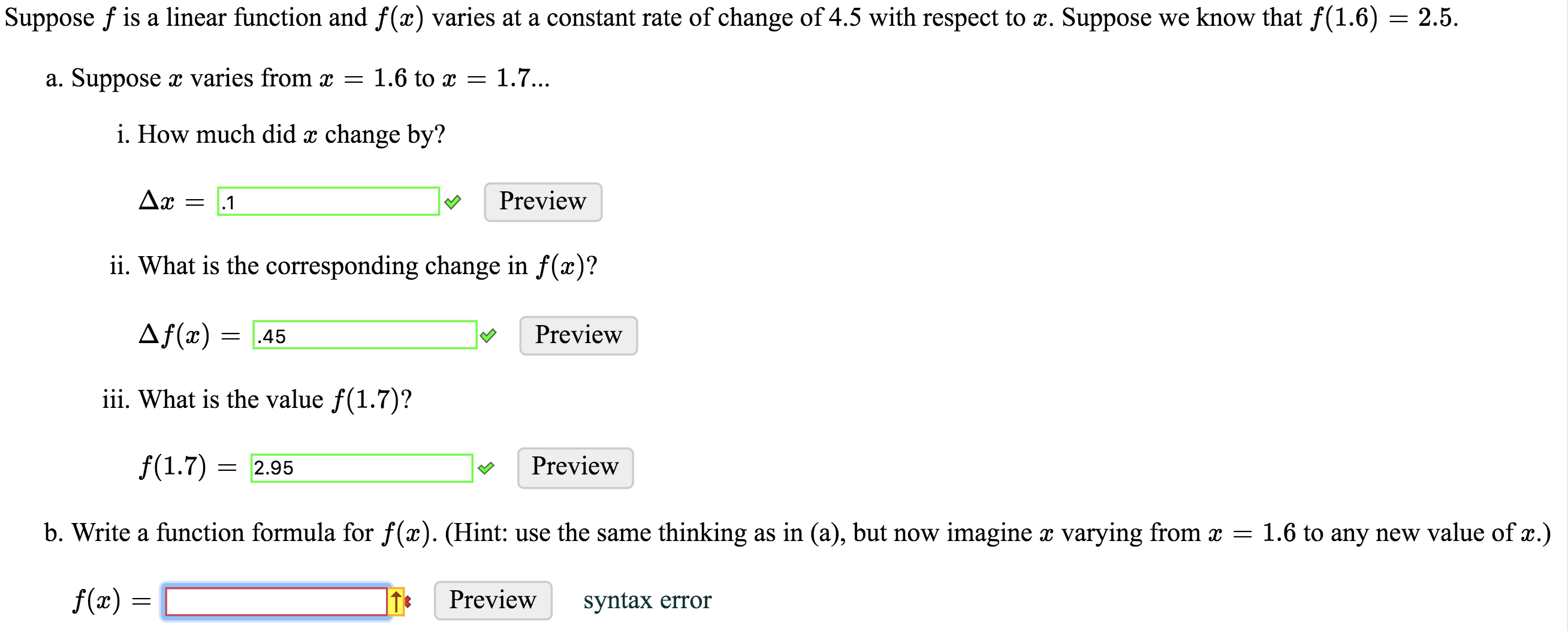# Suppose f is a linear function and f(x) varies at a constant rate of change of 4.5 with respect to x. Suppose we know that f(1.6) = 2.5.varies from x = 1.6 to x1.7...a. Supposei. How much did x change by?Preview.1ii. What is the corresponding change in f(x)?Af(x)Preview.45iii. What is the value f(1.7)?f(1.7)Preview=2.95b. Write a function formula for f(x). (Hint: use the same thinking as in (a), but now imagine x varying from x = 1.6 to any new value of x.)f(x)Previewsyntax error

Question

Please respond to the red boxhelp_outlineImage TranscriptioncloseSuppose f is a linear function and f(x) varies at a constant rate of change of 4.5 with respect to x. Suppose we know that f(1.6) = 2.5. varies from x = 1.6 to x 1.7... a. Suppose i. How much did x change by? Preview .1 ii. What is the corresponding change in f(x)? Af(x) Preview .45 iii. What is the value f(1.7)? f(1.7) Preview =2.95 b. Write a function formula for f(x). (Hint: use the same thinking as in (a), but now imagine x varying from x = 1.6 to any new value of x.) f(x) Preview syntax error fullscreen
check_circleExpert Solution
Step 1

Given, function f is a linear function. Function is varying at constant rate of change 4.5 which means

Step 2

Let us assume

Step 3

Plugging the value of funct...

### Want to see the full answer?

See Solution

#### Want to see this answer and more?

Solutions are written by subject experts who are available 24/7. Questions are typically answered within 1 hour*

See Solution
*Response times may vary by subject and question
Tagged in

### Equations and In-equations